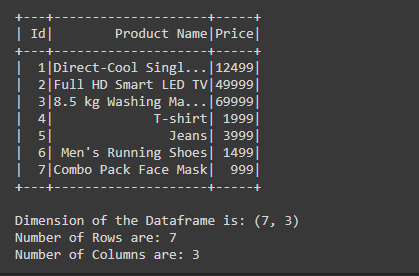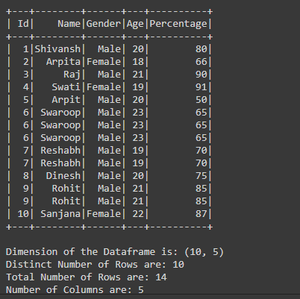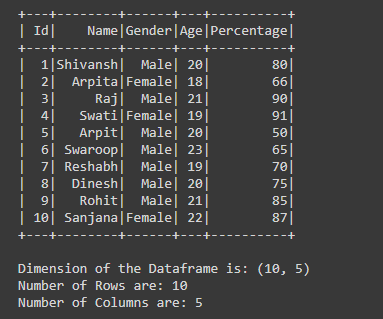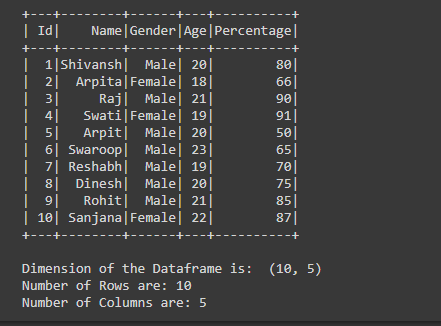Get number of rows and columns of PySpark dataframe

• Last Updated : 13 Sep, 2021

In this article, we will discuss how to get the number of rows and the number of columns of a PySpark dataframe. For finding the number of rows and number of columns we will use count() and columns() with len() function respectively.

• df.count(): This function is used to extract number of rows from the Dataframe.
• df.distinct().count(): This functions is used to extract distinct number rows which are not duplicate/repeating in the Dataframe.
• df.columns(): This function is used to extract the list of columns names present in the Dataframe.
• len(df.columns): This function is used to count number of items present in the list.

Example 1: Get the number of rows and number of columns of dataframe in pyspark.

Python

 # importing necessary librariesfrom pyspark.sql import SparkSession # function to create SparkSessiondef create_session():  spk = SparkSession.builder \      .master("local") \      .appName("Products.com") \      .getOrCreate()  return spk # function to create Dataframedef create_df(spark,data,schema):  df1 = spark.createDataFrame(data,schema)  return df1 # main functionif __name__ == "__main__":   # calling function to create SparkSession  spark = create_session()       input_data = [(1,"Direct-Cool Single Door Refrigerator",12499),          (2,"Full HD Smart LED TV",49999),          (3,"8.5 kg Washing Machine",69999),          (4,"T-shirt",1999),          (5,"Jeans",3999),          (6,"Men's Running Shoes",1499),          (7,"Combo Pack Face Mask",999)]   schm = ["Id","Product Name","Price"]   # calling function to create dataframe  df = create_df(spark,input_data,schm)  df.show()   # extracting number of rows from the Dataframe  row = df.count()     # extracting number of columns from the Dataframe  col = len(df.columns)   # printing  print(f'Dimension of the Dataframe is: {(row,col)}')  print(f'Number of Rows are: {row}')  print(f'Number of Columns are: {col}')

Output:Explanation:

• For counting the number of rows we are using the count() function df.count() which extracts the number of rows from the Dataframe and storing it in the variable named as ‘row’
• For counting the number of columns we are using df.columns() but as this function returns the list of columns names, so for the count the number of items present in the list we are using len() function in which we are passing df.columns() this gives us the total number of columns and store it in the variable named as ‘col’.

Example 2: Getting the Distinct number of rows and columns of Dataframe.

Python

 # importing necessary librariesfrom pyspark.sql import SparkSession # function to create SparkSessiondef create_session():  spk = SparkSession.builder \      .master("local") \      .appName("Student_report.com") \      .getOrCreate()  return spk # function to create Dataframedef create_df(spark,data,schema):  df1 = spark.createDataFrame(data,schema)  return df1 # main functionif __name__ == "__main__":   # calling function to create SparkSession  spark = create_session()       input_data = [(1,"Shivansh","Male",20,80),          (2,"Arpita","Female",18,66),          (3,"Raj","Male",21,90),          (4,"Swati","Female",19,91),          (5,"Arpit","Male",20,50),          (6,"Swaroop","Male",23,65),          (6,"Swaroop","Male",23,65),          (6,"Swaroop","Male",23,65),          (7,"Reshabh","Male",19,70),          (7,"Reshabh","Male",19,70),          (8,"Dinesh","Male",20,75),          (9,"Rohit","Male",21,85),          (9,"Rohit","Male",21,85),          (10,"Sanjana","Female",22,87)]   schm = ["Id","Name","Gender","Age","Percentage"]   # calling function to create dataframe  df = create_df(spark,input_data,schm)  df.show()   # extracting number of distinct rows  # from the Dataframe  row = df.distinct().count()     # extracting total number of rows from  # the Dataframe  all_rows = df.count()     # extracting number of columns from the  # Dataframe  col = len(df.columns)   # printing  print(f'Dimension of the Dataframe is: {(row,col)}')  print(f'Distinct Number of Rows are: {row}')  print(f'Total Number of Rows are: {all_rows}')  print(f'Number of Columns are: {col}')

Output:Explanation:

• For counting the number of distinct rows we are using distinct().count() function which extracts the number of distinct rows from the Dataframe and storing it in the variable named as ‘row’
• For counting the number of columns we are using df.columns() but as this functions returns the list of column names, so for the count the number of items present in the list we are using len() function in which we are passing df.columns() this gives us the total number of columns and store it in the variable named as ‘col’

Example 3: Getting the number of columns using dtypes function.

In the example, after creating the Dataframe we are counting a number of rows using count() function and for counting the number of columns here we are using dtypes function. Since we know that dtypes function returns the list of tuples that contains the column name and datatype of the columns. So for every column, there is the tuple that contains the name and datatype of the column, from the list we are just counting the tuples The number of tuples is equal to the number of columns so this is also the one way to get the number of columns using dtypes function.

Python

 # importing necessary librariesfrom pyspark.sql import SparkSession # function to create SparkSessiondef create_session():  spk = SparkSession.builder \      .master("local") \      .appName("Student_report.com") \      .getOrCreate()  return spk # function to create Dataframedef create_df(spark,data,schema):  df1 = spark.createDataFrame(data,schema)  return df1 # main functionif __name__ == "__main__":   # calling function to create SparkSession  spark = create_session()       input_data = [(1,"Shivansh","Male",20,80),          (2,"Arpita","Female",18,66),          (3,"Raj","Male",21,90),          (4,"Swati","Female",19,91),          (5,"Arpit","Male",20,50),          (6,"Swaroop","Male",23,65),          (7,"Reshabh","Male",19,70),          (8,"Dinesh","Male",20,75),          (9,"Rohit","Male",21,85),          (10,"Sanjana","Female",22,87)]   schm = ["Id","Name","Gender","Age","Percentage"]   # calling function to create dataframe  df = create_df(spark,input_data,schm)  df.show()   # extracting number of rows from the Dataframe  row = df.count()  # extracting number of columns from the Dataframe using dtypes function  col = len(df.dtypes)     # printing  print(f'Dimension of the Dataframe is: {(row,col)}')  print(f'Number of Rows are: {row}')  print(f'Number of Columns are: {col}')

Output:Example 4: Getting the dimension of the PySpark Dataframe by converting PySpark Dataframe to Pandas Dataframe.

In the example code, after creating the Dataframe, we are converting the PySpark Dataframe to Pandas Dataframe using toPandas() function by writing df.toPandas(). After converting the dataframe we are using Pandas function shape for getting the dimension of the Dataframe. This shape function returns the tuple, so for printing the number of row and column individually.

Python

 # importing necessary librariesfrom pyspark.sql import SparkSession # function to create SparkSessiondef create_session():  spk = SparkSession.builder \      .master("local") \      .appName("Student_report.com") \      .getOrCreate()  return spk # function to create Dataframedef create_df(spark,data,schema):  df1 = spark.createDataFrame(data,schema)  return df1 # main functionif __name__ == "__main__":   # calling function to create SparkSession  spark = create_session()       input_data = [(1,"Shivansh","Male",20,80),          (2,"Arpita","Female",18,66),          (3,"Raj","Male",21,90),          (4,"Swati","Female",19,91),          (5,"Arpit","Male",20,50),          (6,"Swaroop","Male",23,65),          (7,"Reshabh","Male",19,70),          (8,"Dinesh","Male",20,75),          (9,"Rohit","Male",21,85),          (10,"Sanjana","Female",22,87)]   schm = ["Id","Name","Gender","Age","Percentage"]   # calling function to create dataframe  df = create_df(spark,input_data,schm)  df.show()   # converting PySpark df to Pandas df using  # toPandas() function  new_df = df.toPandas()     # using Pandas shape function for getting the  # dimension of the df  dimension = new_df.shape   # printing  print("Dimension of the Dataframe is: ",dimension)  print(f'Number of Rows are: {dimension}')  print(f'Number of Columns are: {dimension}')

Output:Attention geek! Strengthen your foundations with the Python Programming Foundation Course and learn the basics.

To begin with, your interview preparations Enhance your Data Structures concepts with the Python DS Course. And to begin with your Machine Learning Journey, join the Machine Learning - Basic Level Course

My Personal Notes arrow_drop_up